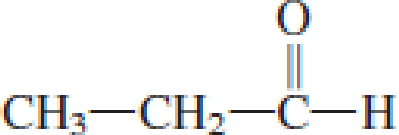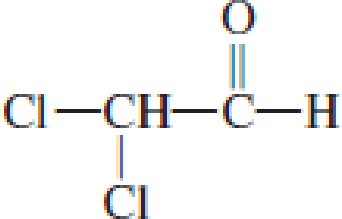Chapter 4, Problem 4.28EP### Organic And Biological Chemistry

7th Edition
STOKER + 1 other
ISBN: 9781305081079

#### Solutions

Chapter
Section### Organic And Biological Chemistry

7th Edition
STOKER + 1 other
ISBN: 9781305081079
Textbook Problem

# Assign a common name to each of the following aldehydes.a. CH3─CH2─CH2─CHO(a)

Interpretation Introduction

Interpretation:

Common name for the given aldehyde has to be given.

Concept Introduction:

Common names for aldehyde are a single word and not like alcohol or ether which contains two words.  The counting of carbon atoms leads to the naming of aldehyde.  From the total number of carbon atoms present in the given aldehyde, the common name of the aldehyde can be derived.  For the counting of 1 to 4 carbon atoms, the name involves form-, acet-, propion-, and butyr- as prefix.

For compounds that contain benzene ring, the common name is obtained as derivatives of benzaldehyde.

Explanation

Given structure of aldehyde is,

The longest carbon chai...

(b)

Interpretation Introduction

Interpretation:

Common name for the given aldehyde has to be given.

Concept Introduction:

Common names for aldehyde are a single word and not like alcohol or ether which contains two words.  The counting of carbon atoms leads to the naming of aldehyde.  From the total number of carbon atoms present in the given aldehyde, the common name of the aldehyde can be derived.  For the counting of 1 to 4 carbon atoms, the name involves form-, acet-, propion-, and butyr- as prefix.

For compounds that contain benzene ring, the common name is obtained as derivatives of benzaldehyde.

(c)

Interpretation Introduction

Interpretation:

Common name for the given aldehyde has to be given.

Concept Introduction:

Common names for aldehyde are a single word and not like alcohol or ether which contains two words.  The counting of carbon atoms leads to the naming of aldehyde.  From the total number of carbon atoms present in the given aldehyde, the common name of the aldehyde can be derived.  For the counting of 1 to 4 carbon atoms, the name involves form-, acet-, propion-, and butyr- as prefix.

For compounds that contain benzene ring, the common name is obtained as derivatives of benzaldehyde.

(d)

Interpretation Introduction

Interpretation:

Common name for the given aldehyde has to be given.

Concept Introduction:

Common names for aldehyde are a single word and not like alcohol or ether which contains two words.  The counting of carbon atoms leads to the naming of aldehyde.  From the total number of carbon atoms present in the given aldehyde, the common name of the aldehyde can be derived.  For the counting of 1 to 4 carbon atoms, the name involves form-, acet-, propion-, and butyr- as prefix.

For compounds that contain benzene ring, the common name is obtained as derivatives of benzaldehyde.

### Still sussing out bartleby?

Check out a sample textbook solution.

See a sample solution

#### The Solution to Your Study Problems

Bartleby provides explanations to thousands of textbook problems written by our experts, many with advanced degrees!

Get Started